# Keeping in Step

## OBJECTIVE: To learn how to divide decimals

In previous lessons, you learned how to ADD AND SUBTRACT DECIMALS and how to MULTIPLY DECIMALS. Lining 'Em Up: https://www.geogebra.org/m/xe7bhcvz Playing Hide and Seek: https://www.geogebra.org/m/hk7yfevm In this lesson, you're going to learn how to DIVIDE DECIMALS. VOCABULARY Dividend—the number to be divided (inside the division box, numerator of a fraction, before the division symbol) Divisor—the number to divide by (outside the division box, denominator of a fraction, after the division symbol) Quotient—the result of division Follow these steps in dividing decimals: Step 1. If the divisor is a decimal, convert it to a whole number by moving the decimal point to the very end of the number. Step 2. Move the decimal point of the dividend to the right the same number of places as the divisor. Fill in any empty spaces that will result from moving the decimal point, if any. Step 3. Convert to long division format (using the division box), if the problem is not yet in that format. Step 4. Perform the division, making sure the decimal point of the quotient is aligned with the new position of decimal point of the dividend. Example 1: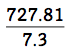Steps 1 and 2. Move the decimal point one place to the right for both numerator and denominator.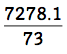Step 3. Change to a division box.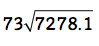Step 4. Solve and align the decimal point. The answer is given up to 2 decimal places.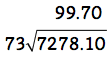Example 2: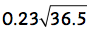Steps 1 and 2. Move the decimal point two places to the right for both dividend and divisor. Affix a zero to the dividend to fill in the empty space.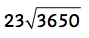Step 3. Skip this step since the problem is already in the proper format. Step 4. Solve and align the decimal point. The answer is given up to 2 decimal places.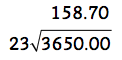Below is a set of problems that require you to divide decimals.

## In this lesson, you learned how to divide decimals.

In future lessons, you'll discover more about fractional parts. Hope you ENJOYED today's lesson!